# SAT Math Multiple Choice Question 987: Answer and Explanation

### Test Information

Question: 987

7.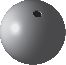A jeweler makes beads for a bracelet by melting silver into spherical molds. Eachmold has a diameter of 1 centimeter. After the silver hardens, the jeweler drillsa small cylindrical hole through the center of the bead for the chain as shown. Thedrill bit has a diameter of 4 millimeters. If the jeweler strings a total of 15 beadson an elastic band, what is the approximate volume of silver, in cubic centimeters,on the bracelet? (Note: There are 10 millimeters in 1 centimeter.)

Note: The volume is approximate because the top and bottom of the cylindrical piece that is drilled out is slightlycurved.

• A.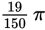• B.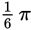• C.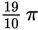• D.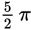Explanation:

C

Difficulty: Hard

Category: Additional Topics in Math / Geometry

Strategic Advice: Staying organized is the key to answering a question like this. Write down what you need to find in words, and then apply the math. Take note that the answers are given as fractions of π, so don't spend time converting numbers to decimals.

Getting to the Answer: You need to find the volume of one bead (after the hole has been drilled) and then multiply this volume by the number of beads (15). Write down something like this: Volume of bead minus volume of drilled-out part = volume of sphere minus volume of cylinder. Check the formula page and be sure to label dimensions as you go. Both formulas involve a radius, but the radii are not the same for the bead and the drilled-out part.

Volume of bead = volume of sphere - volume of cylinder translates to: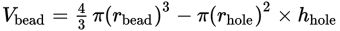At this point, you should jot down the value of each variable in the equation based on the information provided in the question: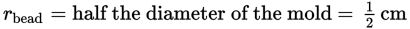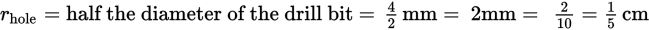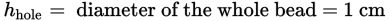Now, carefully substitute each value into the equation and simplify: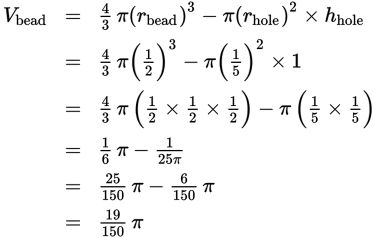Don't forget to multiply this by 15 (because there are 15 beads). The final volume is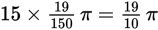, which matches (C).